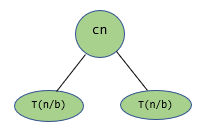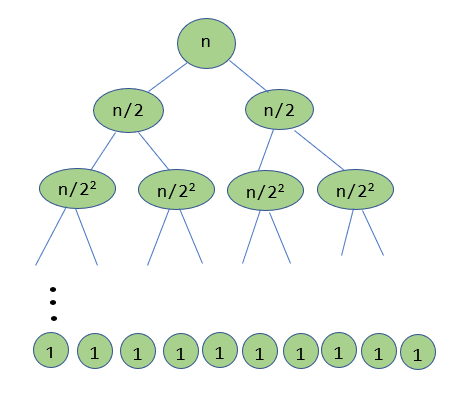×

Search anything:

# Recurrence Tree Method for Time Complexity

#### Time Complexity AlgorithmsIn this article, we have explored Recurrence Tree Method for calculating Time Complexity of different algorithms.

Contents

1. Introduction
2. Recursion Tree Method
3. Example
4. Where to use Recursion Tree Method

## Introduction

A recurrence is an equation or inequality that describes a function in terms of its value on smaller inputs. There are multiple types of recurrences (or recurrence relations), such as linear recurrence relation and divide and conquer recurrence relations. An example of a recurrence relation is given below:

T(n) = 2T(n/2) + cn

Once the recurrence relation of a particular solution is obtained, it remains to solve this relation to obtain the time complexity of the solution. There are multiple ways to solve these relations, which include the subsitution method, the iteration method, the recursion tree method and the master method. We shall explore the recursion tree method in detail.

## Recursion Tree Method

The recursion tree method is commonly used in cases where the problem gets divided into smaller problems, typically of the same size. A recurrence tree is drawn, branching until the base case is reached. Then, we sum the total time taken at all levels in order to derive the overall time complexity.

For example, consider the following example:

T(n) = aT(n/b) + cn

Here, the problem is getting split into a subproblems, each of which has a size of n/b. Hence, the first level of the recurrence tree would look as follows:Here, the topmost level now has a cost of cn, since that was the cost defined in the recurrence relation to split the problem.

This process of splitting the problem into a smaller size continues until a pre-defined base condition is obtained, or the size of the input becomes 1.

## Example

Example: T(n) = 2T(n/2) + n

In this problem, one can observe that the problem is getting split into two problems of half the initial size. Further the additional cost here equals the size. Hence, after the first division, the recursion tree will have two child nodes with input size n/2. We then proceed in this manner until the final input size becomes 1. Therefore, the final recursion tree will look as follows (note that each node contains only the extra cost that is taken) :As the recursion tree is complete, it remains to calculate the total sum of the entries. For that, we first need to determine the number of levels in the recursion tree. Since each level of the tree splits each of the nodes in that level to half the size of their parents, one can conclude that the total number of levels here is log2n.

The next thing we note here is that in each level, the sum of the nodes is n. Therefore, the overall time complexity is given by:

T(n) = n + n + .... log2n times
= n ( 1 + 1 + ....log2n times)
= n log2n
= θ(n log2n)

Therefore, the overall time complexity of the operation with the given recurrence equation is given by θ(n log2n).

## Where to use Recurrence Tree Method

The recurrence tree method is most useful when the recurrence relation splits the given problem into subproblems of uniform size. In this case, drawing conclusions from the recursion tree is an easy task. This is most often true in cases of divide and conquer problems.

However, if the size of the subproblem does not follow a specific pattern, then it becomes tedious to draw the recursion tree, and hence other methods might be preferred. A recursion tree would also not be ideal in case of a linear recursion problem, since each level of the recursion tree would have just one level.

With this article at OpenGenus, you must have the complete idea of Recurrence Tree Method for Time Complexity.#### Aravind Mohandas

Aravind Mohandas is a Master's student at Indian Institute of Technology, Ropar with B.Sc in Computer Science from Christ University, Bangalore. He is an intern at OpenGenus.

Recurrence Tree Method for Time Complexity